• ## K-mean(多维度)聚类算法(matlab代码)

万次阅读 多人点赞 2017-05-26 00:01:57
申明: 仅个人小记 目录  1....     (1) 二维度效果图 ...    (2) 三维度效果图 ...    (3) 多维度k-mean代码     (4) 功能使用示范代码  4. 小结 一 . 效果演示   1. 二维度   ...
申明: 仅个人小记。 Email: officeforcsdn@163.com

目录
效果演示二维度三维度
k-mean 算法思想简要说明代码分析二维度k-mean代码三维度k-mean代码多维度k-mean代码功能使用示范后期函数接口改造 (借助matlab中cell结构实现)新的函数接口使用范例
小结

效果演示
二维度
(1) K = 6; 参与元素个数为1000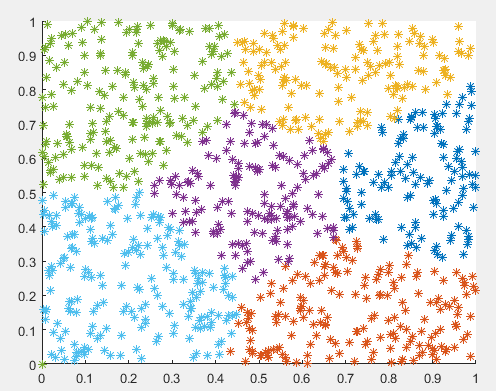(2) K = 7; 参与元素个数为1000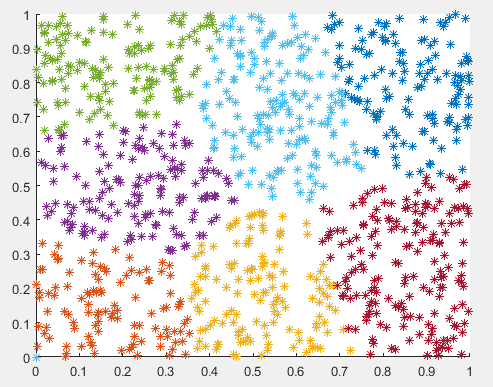三维度
(1)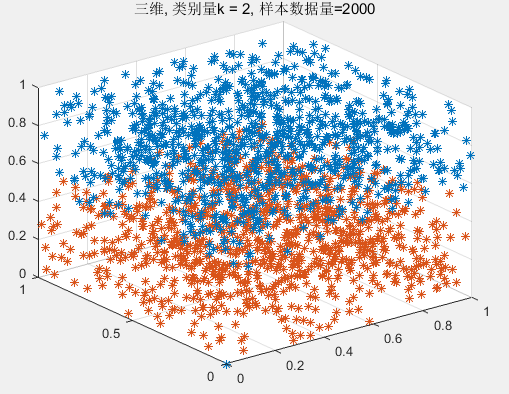(2)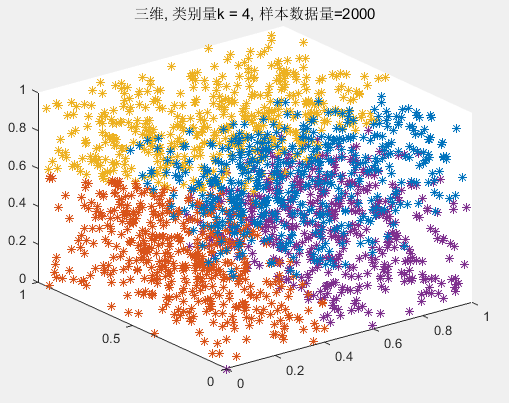k-mean 算法思想简要说明
给定1000个二维坐标点，现在指定要把它们分为k类，面对这样的情况该怎么处理？能想到的思路可能是穷举法，规规矩矩的从这1000个二维坐标点覆盖的二维面积中挑出k个点(我们称之为种子元素)，然后遍历所有的元素点(这里指的就是1000个给定的点）,对每一个元素进行判定类别，即判定每一个元素应该是归为k个类中哪一个类，并把该元素加入在被判定的类中。判断的规则就是，当前元素和k个种子之间的欧几里得距离最短的那个种子所代表的类就是当前元素应该加入的类。所有的元素遍历完毕，我们这是得到K组元素集合，即原来的元素被划分为k类了。这是我们计算每一组的方差，我目前认为应该是不停的用上述方法，得到分类，然后计算方差，最终，要找到每一个类方差最小的情况，这是认为是最优的分类，分类完毕。 显然，上述最大的弊端就是计算量很大，一点优化的成分都没有。k-mean算法思想中提出：但我们得到k组类别集合，计算k组每一组的中心点(方法就是计算均值点)，每一组都得到中心点。这时，我们认为k组中心点为新的种子点。如果本次计算得到的种子点和之前的种子点是完全一致的，那么说明我们已经分类完毕了。如果不一致，如果不一致，我们就会基于的到的新的种子，再进行上述的操作，知道新计算的种子点和上一次的种子点完全匹配。另外，提一下关于第一次选择种子点的问题，应该是第一次选择的种子应该是来源于源数据样本元素(写代码的时候发现有必要这样做，如果不这样，在结果中会出现某一个类别的成员数量为0，显然，这是不合理的。成员个数为0的类别意味着这个类别是不存在，显然是不符合要分为k类的要求)。
代码分析
二维度k-mean代码
function [ resX,resY,record] = FunK_mean( x,y,k )
% 功能：
%     实现k-mean聚类算法
% 输入：
%     二维数据，分别用x,y两个一维向量代表两个维度
%     k 是分成的类别的数量
% 输出：
%     k行的两个矩阵
%     对应同样的第n行，存放着第n类的所有元素
%     record: 记录着每一行的有效元素的个数

j = 1;
% 下面是预分配一些空间
% seedX 和 seedY 中存放着所有种子
seedX = zeros(1,k);
seedY = zeros(1,k);
oldSeedX = zeros(1,k);
oldSeedY = zeros(1,k);
resX = zeros(k,length(x));
resY = zeros(k,length(x));
% 用来记录resX中每一行有效元素的个数
record = zeros(1,k);
for i = 1:k % 产生k个随机种子, 注意： 随机种子是来自元素集合
seedX(i) = x(round(rand()*length(resX)));
seedY(i) = y(round(rand()*length(resX)));
% 为保证种子不重叠
if (i > 1 && seedX(i) == seedX(i-1) && seedY(i) == seedY(i-1))
i = i -1; % 重新产生一个种子
end
end
seedX
seedY
while 1
record(:) = 0; % 重置为零
resX(:) = 0;
resY(:) = 0;
for i = 1:length(x) % 对所有元素遍历
% 下面是判断本次元素应该归为哪一类，这里我们是根据欧几里得距离进行类别判定
% k-mean算法认为元素应该归为距离最近的种子代表的类
distanceMin = 1;
for j = 2:k
if (power(x(i)-seedX(distanceMin),2)+power(y(i)-seedY(distanceMin),2))...
> (power(x(i)-seedX(j),2) + power(y(i)-seedY(j),2))
distanceMin = j;
end
end
% 将本次元素点进行类别归并
resX(distanceMin,record(distanceMin)+1) = x(i);
resY(distanceMin,record(distanceMin)+1) = y(i);
record(distanceMin) = record(distanceMin) + 1;
end
oldSeedX = seedX;
oldSeedY = seedY;
% 移动种子至其类中心
record
for i = 1:k
if record(i) == 0
continue;
end
seedX(i) = sum(resX(i,:))/record(i);
seedY(i) = sum(resY(i,:))/record(i);
end

% 如果本次得到的种子和上次的种子一致，则认为分类完毕。

if mean([seedX == oldSeedX seedY == oldSeedY]) == 1 % 这句话所想表达的意思就是 if seedX == oldSeedX && seedY == oldSeedY
break;
end
end

% 下面代码只是对resX,resY所占用的内寸大小进行简单的优化
maxPos = max(record);
resX = resX(:,1:maxPos);
resY = resY(:,1:maxPos);
end


三维度k-mean代码
function [ resX,resY, resZ,record] = FunK_mean3D( x,y,z,k )
% 功能：
%     实现三维空间k-mean聚类算法
% 输入：
%     三维数据，分别用x,y,z两个一维向量代表两个维度
%     k 是分成的类别的数量
% 输出：
%     k行的两个矩阵
%     对应同样的第n行，存放着第n类的所有元素
%     record: 记录着每一行的有效元素的个数

j = 1;
% 下面是预分配一些空间
% seedX 和 seedY 中存放着所有种子
seedX = zeros(1,k);
seedY = zeros(1,k);
seedZ = zeros(1,k);
oldSeedX = zeros(1,k);
oldSeedY = zeros(1,k);
oldSeedZ = zeros(1,k);
resX = zeros(k,length(x));
resY = zeros(k,length(x));
resZ = zeros(k,length(x));
% 用来记录resX中每一行有效元素的个数
record = zeros(1,k);
for i = 1:k % 产生k个随机种子, 注意： 随机种子是来自元素集合
seedX(i) = x(round(rand()*length(resX)));
seedY(i) = y(round(rand()*length(resX)));
seedZ(i) = z(round(rand()*length(resX)));
% 为保证种子不重叠
if (i > 1 && seedX(i) == seedX(i-1) && seedY(i) == seedY(i-1) && seedZ(i) == seedZ(i-1))
i = i -1; % 重新产生一个种子
end
end

while 1
record(:) = 0; % 重置为零
resX(:) = 0;
resY(:) = 0;
resZ(:) = 0;
for i = 1:length(x) % 对所有元素遍历
% 下面是判断本次元素应该归为哪一类，这里我们是根据欧几里得距离进行类别判定
% k-mean算法认为元素应该归为距离最近的种子代表的类
distanceMin = 1;
for j = 2:k
if (power(x(i)-seedX(distanceMin),2)+power(y(i)-seedY(distanceMin),2)+power(z(i)-seedZ(distanceMin),2))...
> (power(x(i)-seedX(j),2) + power(y(i)-seedY(j),2)+power(z(i)-seedZ(j),2))
distanceMin = j;
end
end
% 将本次元素点进行类别归并
resX(distanceMin,record(distanceMin)+1) = x(i);
resY(distanceMin,record(distanceMin)+1) = y(i);
resZ(distanceMin,record(distanceMin)+1) = z(i);
record(distanceMin) = record(distanceMin) + 1;
end
oldSeedX = seedX;
oldSeedY = seedY;
oldSeedZ = seedZ;
% 移动种子至其类中心
record
for i = 1:k
if record(i) == 0
continue;
end
seedX(i) = sum(resX(i,:))/record(i);
seedY(i) = sum(resY(i,:))/record(i);
seedZ(i) = sum(resZ(i,:))/record(i);
end

% 如果本次得到的种子和上次的种子一致，则认为分类完毕。

if mean([seedX == oldSeedX seedY == oldSeedY seedZ == oldSeedZ]) == 1 % 这句话所想表达的意思就是 if seedX == oldSeedX && seedY == oldSeedY
break;
end
end

maxPos = max(record);
resX = resX(:,1:maxPos);
resY = resY(:,1:maxPos);
resZ = resZ(:,1:maxPos);
end


多维度k-mean代码
返回值res矩阵内容举例示意图：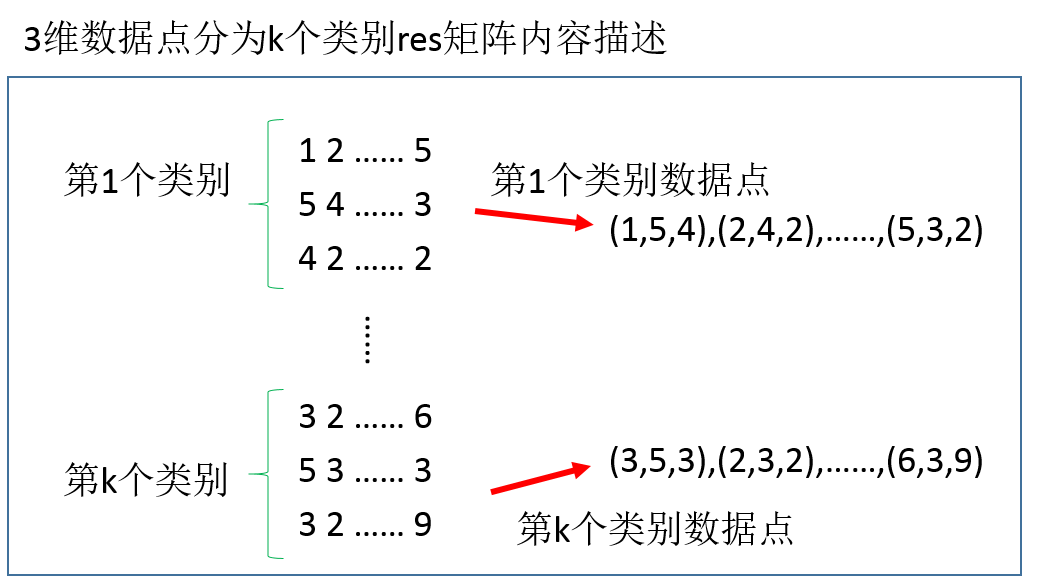function [ res, record] = FunK_meanPolyD(data,k )
% 功能：
%     实现多维空间k-mean聚类算法
% 输入：
%     data是d*n规格的矩阵，其中d代表维度，n代表样本的数量
%     k 是分成的类别的数量
% 输出：
%     res 是行数为(d*k), 列数为record中最大元素值
%	  对于res的行数为d*k的解释：
%		1:d 是对应着第一类别元素
%		d+1:2*d 是对应着第二类别元素
%			···
%		d*(k-1)+1:d*k 是对应着第k类别元素
%
%     record规格为1*k,记录着每一类别的有效元素的个数

j = 1;
% 下面是预分配一些空间
% seedX 和 seedY 中存放着所有种子
[h w] = size(data);
cnt = w; % 输入元素的数量
cntOfDimension = h; % d 中存放着本次处理数据的维度
%seed 中存放种子，每一行代表种子所在的一个维度，每一列是一个种子向量
seed = zeros(cntOfDimension,k);
oldSeed = zeros(cntOfDimension,k);
% 结果矩阵res中，数据存放规则：
%   以d行为一个单位，总共k个d行
%   第一个d行数据存放着第一类元素集合,其他同理
res = zeros(k*cntOfDimension,cnt);
% 用来记录resX中每一行有效元素的个数
record = zeros(1,k);
r = 0;
for i = 1:k % 产生k个随机种子, 注意： 随机种子是来自元素集合
t = round(rand()*cnt);
% 为保证种子不重叠
if i > 1 && t == r
i = i - 1;
continue;
end

seed(:,i) = data(:,t);
r = t;
end

while 1
record(:) = 0; % 重置为零
res(:) = 0;
for i = 1:cnt % 对所有元素遍历
% 下面是判断本次元素应该归为哪一类，这里我们是根据欧几里得距离进行类别判定
% k-mean算法认为元素应该归为距离最近的种子代表的类
distanceMin = 1; % distanceMin 中存放着最短欧几里得距离的种子点的下标
for j = 2:k
% 计算高维度的欧几里得距离
a = 0;
b = 0;
for row = 1:cntOfDimension
a = a + power(data(row,i)-seed(row,distanceMin),2);
b = b + power(data(row,i)-seed(row,j),2);
end
if a > b
distanceMin = j;
end
end
% 将本次元素点进行类别归并
row = (distanceMin-1)*cntOfDimension + 1;
res(row:row+cntOfDimension-1,record(distanceMin)+1) = data(:,i);
record(distanceMin) = record(distanceMin)+1;
end
%record
oldSeed = seed;
% 移动种子至其类中心
for col = 1:k
if record(col) == 0
continue;
end
% 计算新的种子位置
row = (col-1)*cntOfDimension + 1;
seed(:,col) = sum(res(row:row+cntOfDimension-1,:),2)/record(col);
end
% 如果本次得到的种子和上次的种子一致，则认为分类完毕。
if mean(seed == oldSeed) == 1
break;
end
end

maxPos = max(record);
res = res(:,1:maxPos);
end

功能使用示范
% k-mean算法在思想上还是存在弊端的
% k-mean算法是基于欧几里得空间距离进行基本判定的，而实际状况中不一定就是要以欧几里得空间距离作为判断基础的

% 下面是二维k-mean
clear all
close all
t = 1000;%指定样本元素个数
x = rand(1,t);% 产生样本数据
y = rand(1,t);
k = 7;% 指定类别数量
%
[resX,resY,record] = FunK_mean(x,y,k);% record 中存放着每一个类别组的成员数量
% 注意为了编写方便，resX,resY 是以二维矩阵的形式呈现
% resX(i,:) 和resY(i,:) 表示第i个类别组的所有成员，
% 但有效成员的数目不一定等于length(resX(i,:)),而是等于record(i)
% 多余的空位是用零来填充的
hold on
for i = 1:length(record)
plot(resX(i,1:record(i)),resY(i,1:record(i)),'*')
end
% 下面是标记出每一个类别的类别代表点
for i = 1:length(record)
plot(mean(resX(i,1:record(i)),2)',mean(resY(i,1:record(i)),2)','Marker','square','Color','k','MarkerFaceColor','k','LineStyle','none')
end
hold off

% % 三维度k-mean
%
% clear all
% close all
% t = 1000;
% x = rand(1,t);
% y = rand(1,t);
% z = rand(1,t);
% k = 5;
%
% [resX resY resZ record] = FunK_mean3D(x,y,z,k);
%
% for i = 1:length(record)
%     plot3(resX(i,1:record(i)),resY(i,1:record(i)),resZ(i,1:record(i)),'*')
%     hold on
% end
% % 下面是标记出每一个类别的类别代表点
% for i = 1:length(record)
%     plot3(mean(resX(i,1:record(i)),2)',mean(resY(i,1:record(i)),2)',mean(resZ(i,1:record(i)),2)','Marker','square','Color','k','MarkerFaceColor','k','LineStyle','none')
% end

%下面是多维 k-mean演示部分,包括2维，3维度，高维度
clear all
close all
t = 2000;
d = 3;
data = rand(d,t);
k = 5;
[res, record] = FunK_meanPolyD(data,k);

[h, w] = size(res);
if h/k == 2
hold on
for i = 1:k
plot(res(i*2-1,1:record(i)),res(i*2,1:record(i)),'*')
plot(mean(res(i*2-1,1:record(i)),2),mean(res(i*2,1:record(i)),2),'Marker','square','Color','k','MarkerFaceColor','k','LineStyle','none')
end
hold off
elseif h/k == 3
for i = 1:k
plot3(res(i*3-2,1:record(i)),res(i*3-1,1:record(i)),res(i*3,1:record(i)),'*')
plot3(mean(res(i*3-2,1:record(i)),2),mean(res(i*3-1,1:record(i)),2),mean(res(i*3,1:record(i)),2),'Marker','square','Color','k','MarkerFaceColor','k','LineStyle','none')
hold on%注意：hold on 要写在plot3之后，这样三维图形才会正常绘制
end
hold off
else
disp(['结果维度大于3维，不能进行绘制'])
end


后期函数接口改造 (借助matlab中cell结构实现)
function [res_cell, centroid] = FunK_meanPolyD(data,k )
% 输入：
%      data : m * n  m是数据的维度，n是数据的数量
%      k : 类别数量
% 输出：
%     res_cell:  k个cell,每个cell中存放着该类别成员的在data中的下标
%　　 centroid : 每个类别中心点 m*k 格式的矩阵，每一列代表一个类别的中心点
% 下面是预分配一些空间
% seedX 和 seedY 中存放着所有种子
[dim,n] = size(data);
%seed 中存放种子，每一行代表种子所在的一个维度，每一列是一个种子向量
seed = zeros(dim, k);
oldSeed = zeros(dim, k);
res = zeros(k,n);% k 行，一行代表一个类别，每一行存放成员标号(在data中的下标)
record = zeros(1,k);% 用来记录res 中每一行有效成员的个数
res_cell= cell(1,k);

%% 随机初始化种子,这里保证随机种子各不相同
tt = zeros(1,k);
for i = 1:k
flag = 1;
while flag
t = round(rand()*n);
flag = 0;
for j = 1:k
if t == tt(j)
flag = 1;
break;
end
end
end
tt(i) = t;
seed(:,i) = data(:,t);
end

%%  进入kmeans收敛过程
while 1
record(:) = 0; % 重置为零
for i = 1:n % 对所有元素遍历
distMin = 1; % distMin 中存放着最短欧几里得距离的种子点的下标
for j = 2:k
% 计算高维度的欧几里得距离
a = dot(data(:,i)-seed(:,distMin),data(:,i)-seed(:,distMin));
b = dot(data(:,i)-seed(:,j),data(:,i)-seed(:,j));
if a > b
distMin = j;
end
end
record(distMin) = record(distMin)+1;
res(distMin,record(distMin)) = i;
end

oldSeed = seed;
% 计算新的种子节点
seed(:) = 0;
for i = 1:k % 第i个类别
for j = 1:record(i) % 第i个别中的每个成员
seed(:,i) = seed(:,i) + data(:,res(i,j));
end
seed(:,i) = seed(:,i)/record(i); % 计算中心点
end
if seed == oldSeed
% 用cell对最后一次分组结果进行打包
for i =1:k
res_cell{1,i} = res(i,1:record(i));
end
centroid = seed;
break; % 退出kmeans收敛过程
end
end
end

新的函数接口使用范例
close all
x = [1 1 2 2 4 5 5];
y = [1 2 1 3 5 5 3];
k = 2;% 指定类别数量
data = [x; y];
[dim, n] = size(data);
[res_cell,centroid] = FunK_meanPolyD(data,k)

figure;
for i = 1:k
t = res_cell{i};% t 中存放第i类别所有成员在原数据data中的下标
tt = data(:,t); % tt 则是根据t中提供的下标，在data中将第i类别成员全部取出来，放到tt中
if dim == 2 %绘制二维情况
plot(tt(1,:),tt(2,:),'*');
hold on
plot(centroid(1,i),centroid(2,i),'Marker','square','Color','k','MarkerFaceColor','k','LineStyle','none')
elseif dim == 3 % 绘制三维情况
plot3(tt(1,:),tt(2,:),tt(3,:),'*')
hold on
plot3(centroid(1,i),centroid(2,i),centroid(3,i),'Marker','square','Color','k','MarkerFaceColor','k','LineStyle','none')
end
grid on
end

小结
(1) k-mean缺点： 需要指定k，并不能自动合理的对给定的数据进行分类 (2) 优点：思想还是很棒的，尤其是基于新的种子点进一步归类这一思想，这一步骤很好的利用了已经计算过的结果，而不是每一次都是从头开始。 (3) 如果能够自动分类，我觉得也可以这种能力划分到盲源分离领域了。 (4) k-mean算法在思想上还是存在弊端的。我是这样想的：k-mean算法是基于欧几里得空间距离进行基本判定的，而实际状况中不一定就是要以欧几里得空间距离作为判断基础的。这一想法的产生，是因为我想到了数字图像里面RGB空间模型里面，不能用欧几里得距离的大小来衡量两种颜色的相近程度。
展开全文k-mean matlab 盲源分离
• 多对多维度或多值维度 维度表和事实表之间的标准关系是一对多关系，这意味着维度表中的一行记录会连接事实表中的多行记录，但是事实表中的一行记录在维度表中只关联一行记录。这种关系很重要，因为它防止了重复计数...


多对多维度或多值维度
维度表和事实表之间的标准关系是一对多关系，这意味着维度表中的一行记录会连接事实表中的多行记录，但是事实表中的一行记录在维度表中只关联一行记录。这种关系很重要，因为它防止了重复计数。幸运的是，在大多数情况下都是这种一对多关系。 在现实世界中还存在比一对多关系更复杂的两种常见情况： 事实表和维度表之间的多对多关系。 维度表之间的多对多关系。 这两种情况本质是相同的，但事实表和维度表之间的多对多关系少了唯一描述事实和维度组的中间维度。 对于这两种情况，我们介绍一种称为桥接表的中间表，以支持更复杂的多对多关系。
1. 事实表和维度表之间的多对多关系
在多个维度表的值可以赋给单个事实事务时，事实表和维度表之间通常是多对多关系。一个常见的示例是多个销售代表可以参与给定的销售事务，这种情形经常发生在涉及大宗交易的复杂销售事件中(例如计算机系统)。精确地处理这种情况需要创建一个桥接表，将销售代表组合成一个组。SalesRepGroup桥接表如图2-4所示。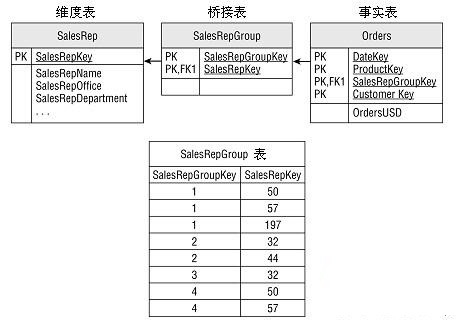ETL过程需要针对每条引入的事实表记录中的销售代表组合，在桥接表中查找相应的销售代表组键。如果该销售代表组键不存在，就添加一个新的销售代表组。注意图2-4所示的桥接表有重复计数的风险。如果按照销售代表累加销售量，那么每个销售代表都会对总销售量做出贡献。对某些分析而言结果是正确的，但对于其他情况仍会有重复计数的问题。要解决这个问题，可以向桥接表中添加加权因子列。加权因子是一个分数值，所有的销售代表组的加权因子累加起来为1。将加权因子和累加事实相乘，以按照每个销售代表在分组中的比重分配事实。 注意可能需要在Orders和SalesRepGroup之间添加一个SalesRepGroupKey表，以支持真正的主键-外键关系。这会把这个事实-维度实例变成维度-维度实例。
2．维度之间的多对多关系
从分析的角度来看，维度之间的多对多关系是一个很重要的概念，大多数维度都不是完全相互独立的。维度之间的独立性是连续的，而不是有或没有这两种截然不同的状态。例如在连续的一端，零售店这条链状关系的库存维度和产品维度是相对独立的，而不是绝对独立的。一些库存方式不适合某些产品。其他维度之间的关系则紧密得多，但是由于存在多对多关系，因此很难将其组合成单个维度。例如在银行系统中，账户和顾客之间有直接关系，但不是一对一的关系。一个账户可以有一个或多个签名确认的顾客，一个顾客也可有多个账户。银行通常从账户的角度来处理数据；MonthAccountSnapshot(月账快照)是金融机构中常见的一种事实表。因为账户和顾客之间存在的多对多关系，这种更多关注账户的系统就很难按照顾客来查看账户。一种方法是创建CustomerGroup桥接表来连接事实表，例如前面多对多示例中的SalesRepGroup表。较好的方法是利用账户和顾客之间的关系，如图2-5所示。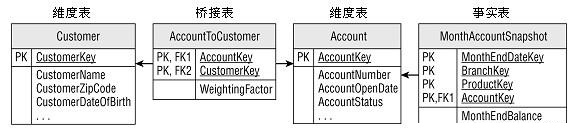账户和顾客维度之间的AccountToCustomer桥接表可以捕获多对多关系，并且有几个显著的优点。首先，源系统中的关系是已知的，因此创建桥接表比手动构建SalesRepGroup维度表更容易。其次，账户-顾客关系自身就非常有趣。AccountToCustomer桥接表可以回答诸如”每个顾客的平均账户数量是多少？”这样的问题，而无须连接任何事实表。 桥接表经常是底层业务过程的标志，特别是在需要跟踪桥接表随时间而产生的变化(即关系本身是类型2变化)时。对顾客和账户来说，业务过程可能称为账户维护，其中一项事务可能称作”添加签名人”。如果3个顾客与同一个账户关联，在源系统中该账户就会有3个”Add(添加)”事务。通常这些事务和它们表示的业务过程还不是很重要，不需要在DW/BI系统中通过它们自身的事实表来跟踪。然而，这些关系和它们产生的变化对分析业务来说是相当重要的。我们在维度模型中把它们包含为渐变维度，在一些情况下包含为桥接表。
                                </div>


展开全文数据仓库
• 针对视频自动描述任务中的复杂信息表征问题，提出一种多维度和多模态视觉特征的提取和融合方法。首先通过迁移学习提取视频序列的静态和动态等多维度特征，并采用图像描述算法提取视频关键帧的语义信息，完成视频信息...迁移学习 长短期记忆网络 循环神经网络
• 针对显式反馈信息作出的推荐在准确率和数据稀疏性处理上还存在缺陷的问题，引入隐式反馈信息，设计和实现了一种引入隐式反馈的多维度推荐算法（iMCF）。该算法涵盖用户、项目和隐式反馈三个维度的信息。对于前两个...
• 针对当前输电线路故障诊断的需求，结合智能电网运行中产生的大量结构多样、来源复杂的数据，将这些大数据归类于不同的维度，设计了基于多维度数据融合的输电线路故障智能诊断系统。对多维度的诊断结果融合架构、融合...
• 精品文档可编辑 值得下载 技工院校多维度教材开发研究 摘 要采用多维度教材开发模式以学生的核心职业素养和核心职业技能的双核培养理念为指导以技能为载体在技能训练中渗透素养教育通过开发多维度主教材多维度辅助...
• 1多维度盈利分析是财务部门职能转型的必然要 求 财务管理职能不断深化与经营活动密切结合要加强基于客户产 品等经营要素的支持服务这时管理会计相应也必须延伸到对客户 产品等经营要素的计量中而利润计量是重要的...
• 针对传统元数据检索过程中的效率低问题，提出了基于多维度的元数据检索算法研究。首先，对元数据和可持续发展元数据结构进行分析。然后，分析信息检索的设计思路设计元数据库，提出元数据信息的资源组织，针对提出的...
• 计算多维度的平方和函数的最小值，个体的维数为10。
• MATLAB工具箱大全-多维度图像分割工具箱AOSLevelsetSegmentationToolboxM
• 针对传统多维度文本聚类算法把文本表示与聚类过程分离，忽略了维度间的互补特性的问题，提出了一种差异互补的迭代式多维度文本聚类算法——CMDC，实现文本聚类与特征调整过程的统一优化。CMDC算法挑选维度聚类间结果...
• 以并网风电与储能系统时空多维度上的耦合互动特性和风火储电力系统间动态协调机制的综合分析为基础，建立考虑风储系统功率传递与能量时空多维度输移特性、储能系统能量周期性循环与功率能量转移守恒规律、风火储系统...
• 多维度盈利性分析?财务管理职能不断深化与经营活动密切结合要加强基于客户产 品等经营要素的支持服务这时管理会计相应也必须延伸到对客户 产品等经营要素的计量中而利润计量是重要的完整的计量在计量 的基础上其他...
• 维度——多对多维度的建立   什么是多对多维度？   我们正常遇到的数据结构是一对一，或者一对多的数据结构，也就是树形结构。但是现实中存在着多对多的结构，比如一笔销售来自一个店铺，但是一个店铺包含多个...
维度——多对多维度的建立

什么是多对多维度？

我们正常遇到的数据结构是一对一，或者一对多的数据结构，也就是树形结构。但是现实中存在着多对多的结构，比如一笔销售来自一个店铺，但是一个店铺包含多个铺位，这时一笔销售就对应着多个铺位，而一个铺位又对应着多笔销售，这样就形成了多对多的关系。

多对多的关系需要用一个关系表来对关系进行维护，如下：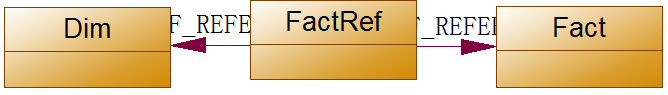1          Dim表建立维度
2          Fact表建立维度DimFact，该维度不关联其它任何维度，由于是用事实表建立维度所以我们成为事实维度
3          建立包含Fact表的cube
4          Cube中添加FactRef表为度量值组
5          Cube的维度用法中添加维度Dim，可以看到Dim和FactRef建立了常规关系
6          Cube的维度用法中添加维度Dim与Fact度量值组间的关系，为多对多关系，中间度量值组为FactRef度量值组
7          执行，查看

展开全文•解决方案
• 多维度对抗Windows_AppLocker 安全开发 安全防御 金融安全 安全众测 法律法规
• 内网-多维度对抗 Windows AppLocker.pdf互联网...